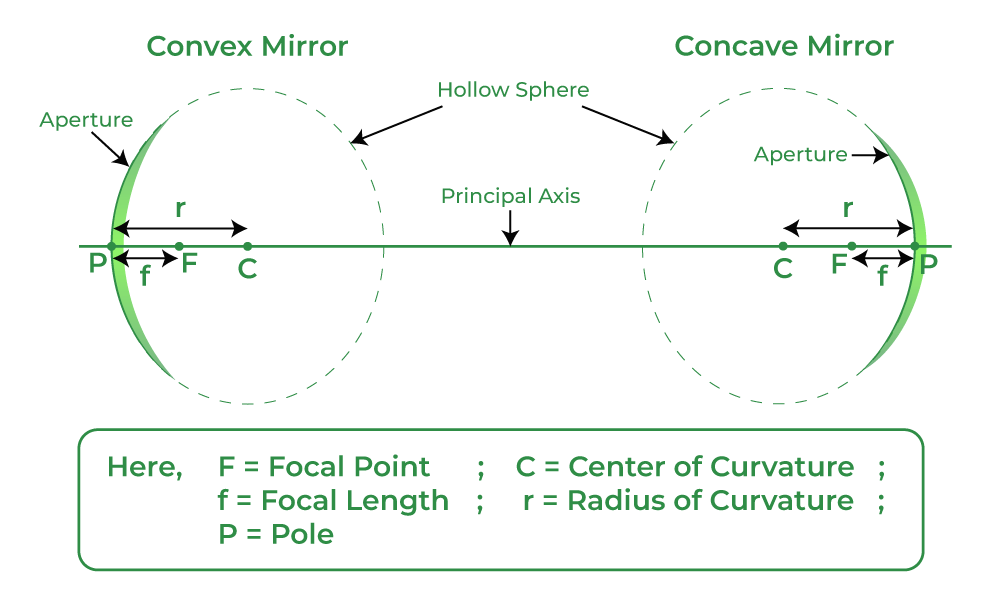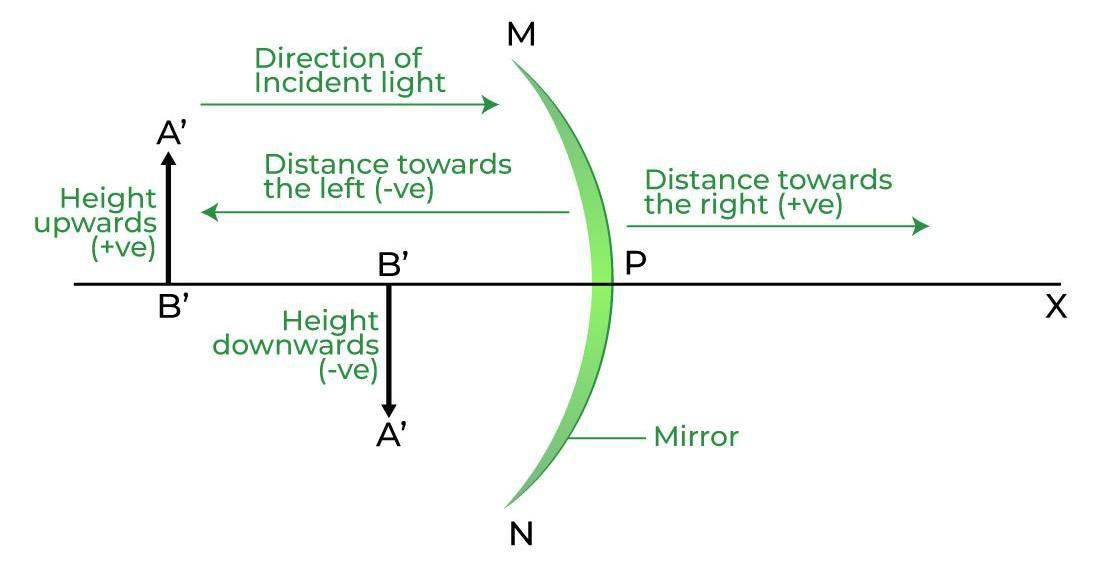GFG App
Open AppBrowser
Continue

# Sign Convention for Spherical Mirrors

While studying the reflection of light by spherical mirrors and the formation of images by spherical mirrors, a set of sign conventions are needed to learn that is required to measure the focal length, the distance of the object or image from the mirror, and the magnification of the mirror.

Before, understanding the concept of sign conventions for spherical mirrors first discuss some commonly used terms in a spherical mirror.

## Commonly Used Terms in Spherical Mirrors### Aperture

The part of a spherical mirror that is exposed to all the light rays that incident on it is called the aperture of the spherical mirror. In other words, the diameter (XY) of the aperture of the concave mirrors and convex mirror is shown in the figure, known as its aperture

### Centre of Curvature

The center of a hollow sphere of which the curved or spherical mirror forms a part is called the center of curvature. It is denoted by C (as shown in the Figure).

The radius of a hollow sphere of which the spherical mirror forms a part is called the radius of curvature. It is denoted by R.

### Pole

The center of the spherical surface is called its pole. It is denoted by P

### Principal Axis

The line joining the center of curvature (C) and pole (P) of a spherical mirror and extended on either side is called the principal axis

### Principal Focus

A point on the principal axis of a spherical mirror where the rays of light parallel to the principal axis meet or appear to meet after reflection from the spherical mirror is called the principal focus. It is denoted by F.

• In the case of a concave mirror, the rays of light parallel to the principal axis after reflection actually meet the principal axis at F as shown in figure 2. So, the principal focus of a concave mirror is real.
• In the case of a convex mirror, the rays of light parallel to the principal axis after reflection appear to meet or diverge from the principal axis at F as shown in figure 3. So, the principal focus of a convex mirror is virtual.

### Focal Plane

A plane normal or perpendicular to the principal axis and passing through the principal focus (F) of a spherical mirror is called the focal plane of the spherical mirror.

### Focal Length

The distance between the pole (P) and principal focus (f) of a spherical mirror is called the focal length of the mirror. It is denoted by f. As shown in the figure above, the focal length of the mirror is represented by PF.

## Sign Convention for Spherical Mirrors

The set of guidelines to set signs for image distance, object distance, focal length, etc for mathematical calculation during an image formation is called the Sign Convention. The sign conventions in the case of the spherical mirrors are made in taking into consideration that the objects are always placed on the left side of the mirror, such that the direction of incident light is from left to right.The sign conventions followed for any spherical mirror are given as:

• All distances are measured from the pole of a spherical error.
• Distances measured in the direction of incident light are taken as positive, while distances measured in a direction opposite to the direction of the incident light are taken as negative.
• The upward distances perpendicular to the principal axis are taken as positive, while the downward distances perpendicular to the principal axis is taken as negative.
• For convenience, the object is assumed to be placed on the left side of a mirror. Hence, the distance of an object from the pole of a spherical mirror is taken as negative.
• Since the incident light always goes from left to right, all the distances measured from the pole (P) of the mirror to the right side will be considered positive (because they will be in the same directions as the incident light). On the other hand, all the distances measured from pole (P) of the mirror to the left  will be negative (because they are measured against the direction of incident light)

## Important Points to Remember

• According to the sign convention, the distances towards the left of the mirror are negative. Since an object is always placed to the left side of a mirror, therefore, the object distance (u) is always negative.
• The images formed by a concave mirror can be either behind the mirror (virtual) or in front of the mirror (real). So, the image distance (v) for a concave mirror can be either positive or negative depending on the position of the image.
• If the image is formed behind a concave mirror, the image distance (v) is positive but if the image is formed in front of the mirror, then the image distance will be negative.
• In a convex mirror, the image is always formed on the right-hand side (behind the mirror), so the image distance (o) for a convex mirror will be always positive.
• The focus of a concave mirror is in front of the mirror on the left side, so the focal length of a concave mirror will be negative (and written with a minus sign, say, -10 cm).
• On the other hand, the focus of the convex mirror is behind the mirror on the right side, so the focal length (and written with a plus sign, say +20 cm or just 20 cm), of a convex mirror is positive.
• The Focal Length and radius of curvature of a concave mirror are taken negatively.
• The Focal Length and radius of curvature of a convex mirror are taken positively.

## Mirror Formula

The distance of the position of an object on the principal axis from the pole of a spherical mirror is known as object distance. It is denoted by u. The distance of the position of the image of an object on the principal axis from the pole of a spherical mirror is known as the image distance. It is denoted by v.

The relation between v and f of a spherical mirror is known as the mirror formula

It is given by,

1 / Object Distance + 1 / Image Distance = 1 / Focal Length

or

1/u + 1/v = 1/f

where

• u is the object distance
• v is the image distance and
• f is the focal length of the mirror.

## Magnification (or Linear magnification)

Linear Magnification produced by a mirror is defined as the ratio of the size (or height) of the image to the size (or height) of the object. It is denoted by m. If h’ is the size (or height) of the image produced by the mirror and h is the size (or height) of the object.

Then, Linear magnification is:

Magnification = Height of Image / Height of Object

or

m = h/ h

where

• m is the magnification of the spherical mirror,
• h’ is the Height of Image, and
• h is the Height of Object.

Linear magnification has no unit.

## Solved Examples on Sign Conventions for Spherical Mirrors

Example 1: A concave mirror produces two times magnified real image of an object placed 10 cm in front of it. Find the position of the image.

Solution:

Here, -10 cm (Sign convention)

m = – 2 ( Image is real).

But m =-v/u

-2=-v/u

v=-20 cm

Thus, image of the object is at 20 cm from the pole of the mirror and in front of the mirror.

Example 2: An object of 5 cm in size is placed at a distance of 20 cm from its concave minor of the focal length of 15 cm At what distance from the mirror, should a screen be placed to get the sharp image? Also, calculate the size of the image.

Solution:

Given that,

h=+5 cm

f = – 15.0 cm          (Sign convention)

u = – 20 cm           (Sign convention)

Determination of the position of image.

Using,

1/u + 1/v =1/f

We get ,

1/v = 1/f-1/u

v = -60cm

So the screen must be placed at a distance of 60 cm in front of the concave mirror.

Determination of size of the image and its nature.

Using,

m= h’/h =-v/u

h=-(v/u)h

= -15 cm

Thus, the size of image -15cm, negative sign with h’ shows that the image is real and inverted

Example 3: A convex mirror used in a bus has a radius of curvature of 3.5 m. If the driver of the bus locates a car 10 m behind the bus, find the position, nature, and size of the image of the car.

Solution:

Here, R = 3-5 m f = R 2 3-5 2 = 1.75m, u = – 100 m.

Determination of the position of the car.

Using, Using, 1/u + 1/v =1/f

1/v =1/f -1/u

1/v= 1/1.75 -1/(-10)

v = 1.5 m

Thus, the car appears to be at 1.5 m from the convex mirror and behind the mirror.

Determination of the size and nature of the image

Using, m= h’/h =-v/u

= -1.5/-10

= 0.15

Thus, the size of the image of the car is 0.15 times the actual size of the car.

Since m is positive, so image of the car is virtual and erect (i.e., upright).

Example 4: Determine the focal length of the concave mirror given the radius of curvature is 20 cm.

Solution:

Given that,

The radius of curvature of the mirror, R is 20 cm.

Using the formula,

R = f/2

or

f = R × 2

= 20 cm × 2

= 40 cm

Hence, the focal length of the mirror is 40 cm.

## FAQs on Sign Conventions for Spherical Mirrors

Question 1: What is the function of a Convex Mirror?

Since, the convex mirror is a diverging mirror. So the light falls on the mirror reflects from it in the outward direction and hence the image formed behind the mirror.

Question 2: Write down the Mirror Formula.

The mirror formula is the relation between the object, image, and focal length of the spherical mirror. The mirror formula is given as,

1/u + 1/v = 1/f

where

• u is the object distance
• v is the image distance and
• f is the focal length of the mirror.

Question 3: What is the function of a Concave Mirror?

Since, the concave mirror is a converging mirror. So the light falls on the mirror reflects from it in the inward direction and hence the image formed in front of the mirror.

Question 4: What is the Sign Convention followed for the Image distance of the Convex Mirror?

In a convex mirror, the image is always formed on the right-hand side (behind the mirror), so the image distance (o) for a convex mirror will be always positive.

Question 5: What is the Sign Convention followed for the Image distance of the Concave Mirror?